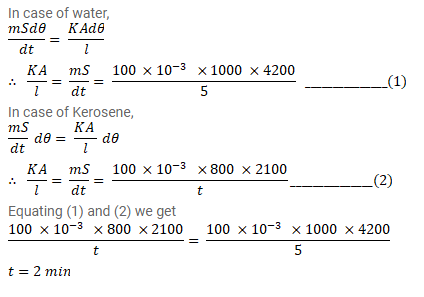# A calorimeter of negligible heat capacity containsQuestion:

A calorimeter of negligible heat capacity contains $100 \mathrm{cc}$ of water at $40^{\circ} \mathrm{C}$. The water cools to $35^{\circ} \mathrm{C}$ in 5 minutes. The water is now replaced by K-oil of equal volume at $40^{\circ} \mathrm{C}$ Find the time taken for the temperature to become $35^{\circ} \mathrm{C}$ under similar conditions. Specific heat capacities of water and K-oil are $4200 \mathrm{~J} / \mathrm{kg}$ - $\mathrm{K}$ and $2100 \mathrm{~J} / \mathrm{kg}-\mathrm{K}$ respectively. Density of K-oil = 800 $\mathrm{kg} / \mathrm{m}^{3}$.

Solution: